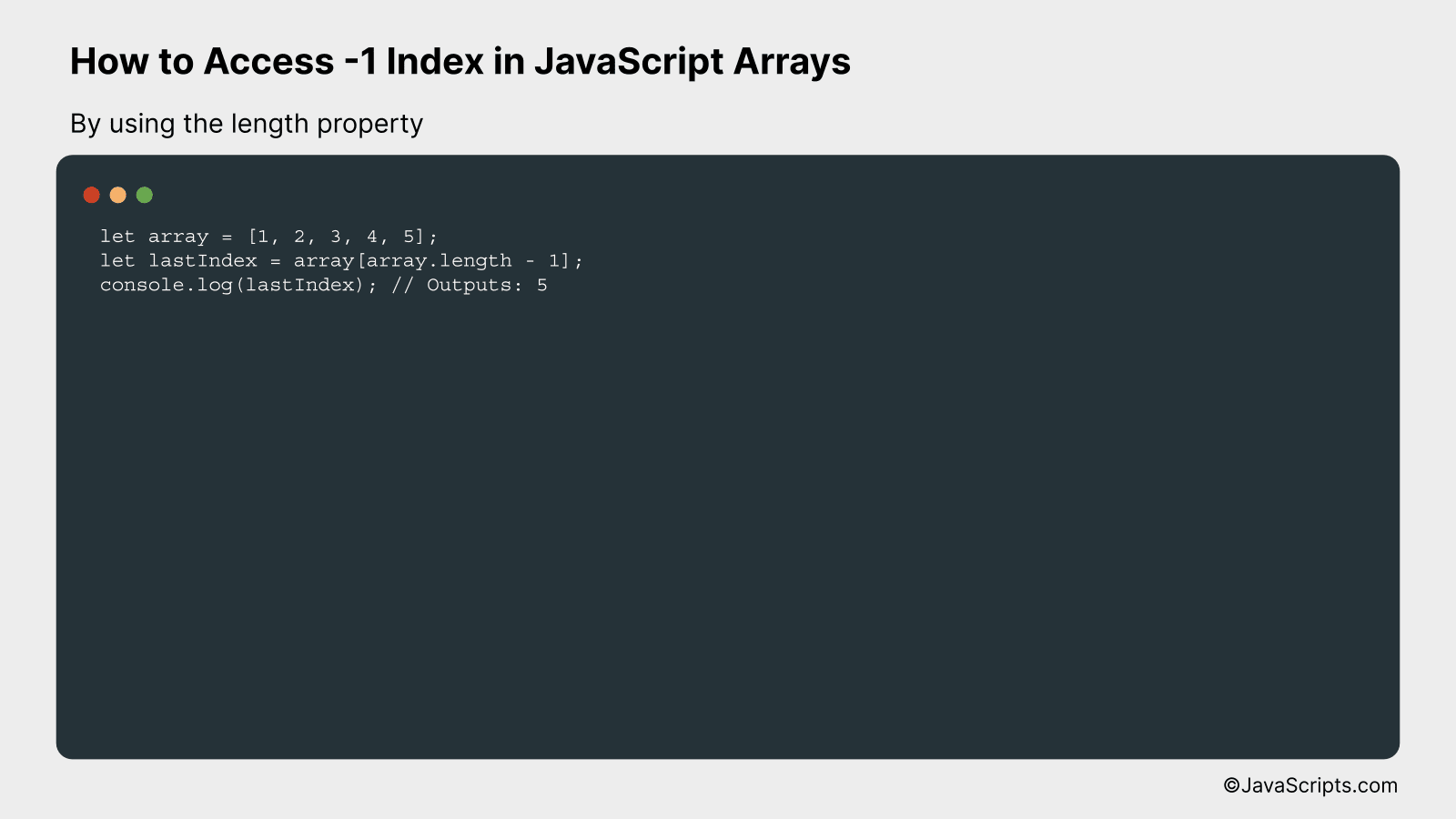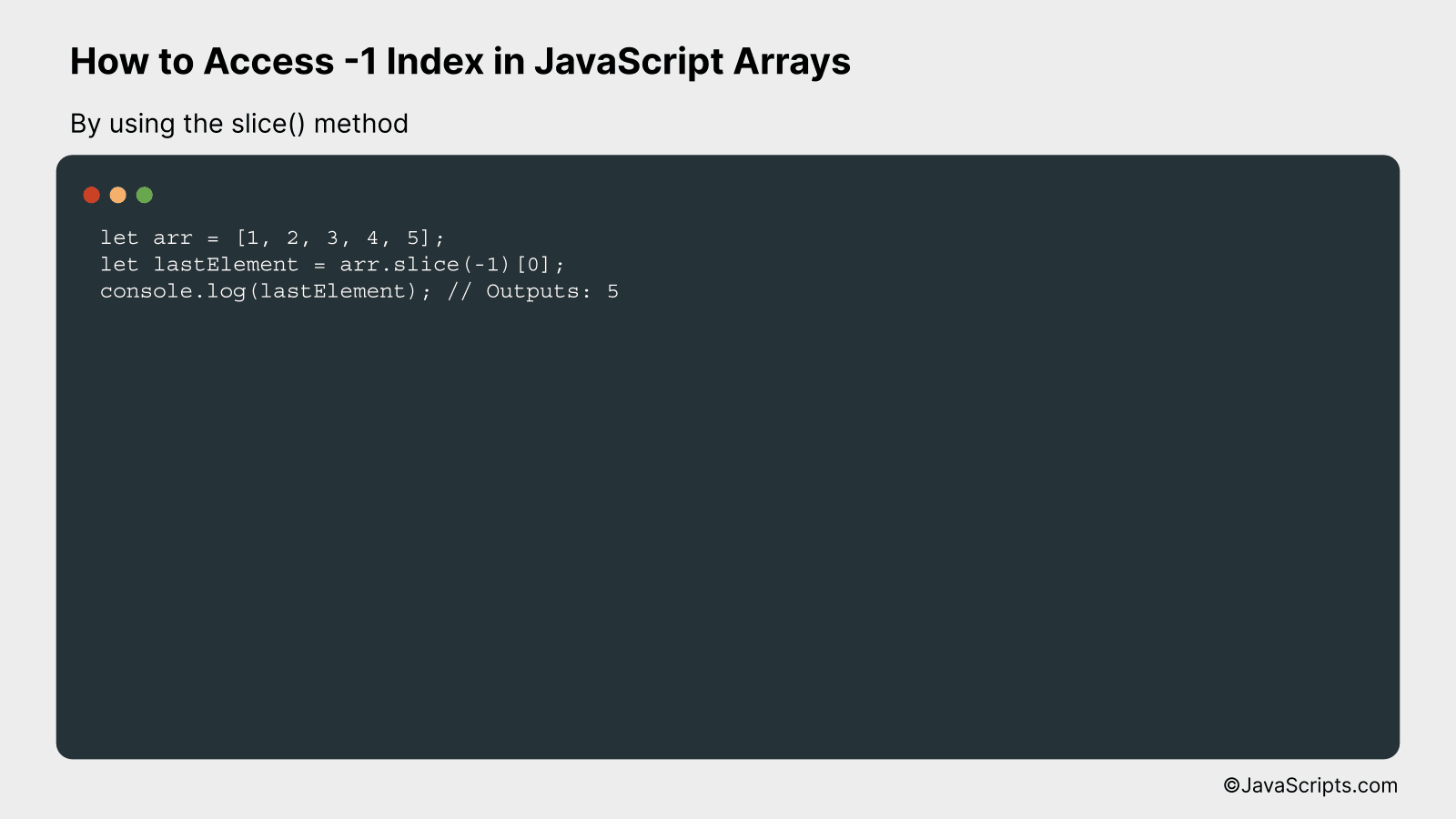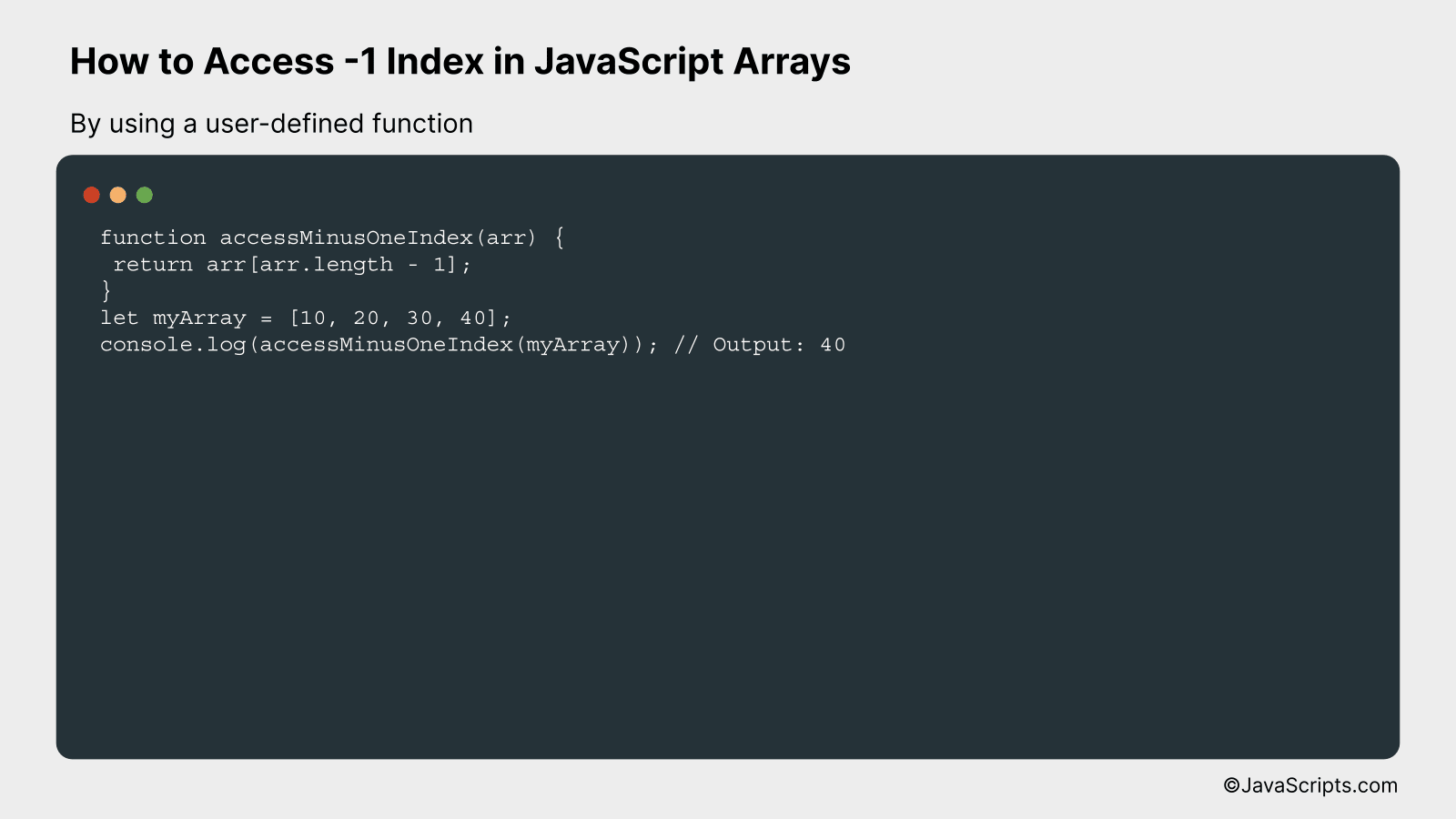# How to Access -1 Index in JavaScript Arrays

Ever found yourself lost in a maze of JavaScript arrays, wishing you could simply jump to the end without traversing through each element one by one? You’re not alone. Many JavaScript developers, especially those new to the language, often overlook the ease of accessing the last element in an array.

Now, imagine if we could just use a handy -1 index to instantly reach the last item? Sounds intriguing, right? Together, let’s explore a smart and simple way to achieve this in JavaScript.

In this post, we will be looking at the following 3 ways to access -1 index in JavaScript arrays:

• By using the length property
• By using the slice() method
• By using a user-defined function

Let’s explore each…

## #1 – By using the length propertyIn JavaScript, accessing the -1 index of an array with the length property will return the last element in the array. This is because arrays in JavaScript are 0-indexed, so the index of the last element is the total length of the array minus one. This can be better understood through the following code example:

``````
let array = [1, 2, 3, 4, 5];
let lastIndex = array[array.length - 1];
console.log(lastIndex); // Outputs: 5
``````

### How it works

The code snippet above uses the ‘length’ property of an array to determine the index of the last element in the array. By subtracting 1 from the length of the array, we can access the last element of that array.

• Step 1: Define an array. In the above example, an array of numbers is defined using the code `let array = [1, 2, 3, 4, 5];`.
• Step 2: Calculate the last index of the array. Since arrays in JavaScript are 0-indexed, the index of the last element is the length of the array minus one. This is done in the code `let lastIndex = array[array.length - 1];`.
• Step 3: Print the last element of the array. This is done using the `console.log()` function with the ‘lastIndex’ variable as the argument.

## #2 – By using the slice() methodIn JavaScript, the slice() method can be used to access the last index (or -1 index) of an array by slicing from the end. The following example demonstrates this technique.

``````
let arr = [1, 2, 3, 4, 5];
let lastElement = arr.slice(-1);
console.log(lastElement); // Outputs: 5
``````

### How it works

The slice() method retrieves a section of an array based on the start and end arguments provided. When a negative value such as -1 is passed, it starts from the end of the array. Hence, slice(-1) retrieves the last element of the array. However, slice(-1) returns an array with the last element, so we use  to access this element.

• Step 1: Initialize an array named ‘arr’ with elements 1, 2, 3, 4, 5.
• Step 2: Apply the slice() method on the ‘arr’ array with the argument as -1. This retrieves a new array with the last element from ‘arr’.
• Step 3: Access the first (and only) element in the new array using the index . This gives us the last element from the ‘arr’ array.
• Step 4: Log this value to the console. The output will be 5, which is the last element of the ‘arr’ array.

## #3 – By using a user-defined functionAccessing a -1 index in a JavaScript array means attempting to access the last element of the array. We can create a user-defined function that will take the array as input and return the last element of the array. Consider an example where we have an array [10, 20, 30, 40]; accessing the -1 index using our user-defined function will return 40.

``````
function accessMinusOneIndex(arr) {
return arr[arr.length - 1];
}
let myArray = [10, 20, 30, 40];
console.log(accessMinusOneIndex(myArray)); // Output: 40
``````

### How it works

The user-defined function ‘accessMinusOneIndex’ takes an array as an argument. Inside the function, we utilize the array’s length property to get the size of the array and then subtract one from that size to access the last element (as arrays in JavaScript are 0-indexed).

• First, we define our user-defined function ‘accessMinusOneIndex’ which takes ‘arr’ as a parameter, which is the array we want to access.
• Inside this function, we use ‘arr.length – 1’ to get the last index of the array. JavaScript arrays being 0-indexed, the last element is at the ‘length – 1’ index.
• We then return this last element of the array from the function.
• To use this function, we create an array ‘myArray’ with values [10, 20, 30, 40].
• We then call our user-defined function ‘accessMinusOneIndex’ with ‘myArray’ as an argument and log the result to the console.
• The output will be 40, which is the last element of the array ‘myArray’.

Related:

In conclusion, it’s clear that JavaScript arrays do not directly support negative indexing. However, we have seen that using a simple trick of subtracting 1 from the array length can help us access the last item.

It’s always helpful to remember that while JavaScript may not have certain inbuilt features, with a little creativity, we can develop our own solutions to overcome these limitations. Stay innovative and keep coding!